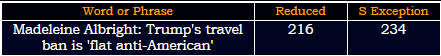## Cipher## Jan 31, 2017

### CNN - Trump travel ban is 'flat anti-American' 227 318 once again

Earlier today I decoded a story with a lot of 318 153 227 connections and CNN released another post with these incredible numbers

If you are new to these numbers check the previous post first - CNN-318-153-bible-reference-with-lucy216 = 6*6*6
234+432 = 666Madeleine = 4+1+4+5+3+5+9+5+5 = 41 (Reduced)
Albright = 1+3+2+9+9+7+8+2 = 41 (Reduced)
13th prime number is 41
The story comes out on 31st day of the year - 1/31 = 32 (32nd prime number is 131)

Her birthday is on 15th of May which is 104 days from nowFifteenth of May = 6+9+6+20+5+5+14+20+8 + 15+6 + 13+1+25 = 153 (Ordinal)
5/15 is 135th day in the year (135+531 = 666)
This story comes from New Day (CNN's TV show)
New day = 40+5+900 + 4+1+400 = 1350 (Jewish)
New day = 84+30+138 + 24+6+150 = 432 (Sumerian)
Their tweet shows 2:34 PM - 234+432 = 666
January Thirty first = 600+1+40+200+1+80+400 + 100+8+9+80+100+400 + 6+9+80+90+100 = 2304 (Jewish)

Madeleine = 30+1+4+5+20+5+9+40+5 = 119 (Jewish) - Saturn = 119 (Francis Bacon)
Albright = 1+20+2+80+9+7+8+100 = 227 (Jewish)
It's very interesting that she has these 2 numbers
119+191+911 = 1221
227+272+722 = 1221

Former Secretary of State Madeleine Albright is slamming President's Trump's travel ban as reckless and "flat anti-American."

"flat anti-American." = 6+3+1+2 + 1+5+2+9+1+4+5+9+9+3+1+5 = 66 (Reduced)
"We are a country that has been created and populated by people from other countries," she told CNN's Chris Cuomo on Tuesday
We are a country that has been created and populated by people from other countries, = 6006 (English)
Thirty three 66 (Reduced)

"We are a country that has been created and populated by people from other countries," = 318 (Reduced)

Just before they end the story they drop another 227 connecting to her last name
"Disruption is interesting, Chris, (but) destruction is bad," she said.

"Disruption is interesting, Chris, (but) destruction is bad," = 227 (Reduced)
Twenty two divided by seven = 314
Seven hundred seventy seven = 314"and there's going to be tit for tat." = 1+5+4 + 2+8+5+9+5+10 + 7+6+9+5+7 + 2+6 + 2+5 + 2+9+2 + 6+6+9 + 2+1+2 = 137 (S Exception)
33rd prime number is 137

Trump signed a 120 day ban "to keep the refugees from entering the country and to ban immigrants from seven predominantly Muslim nations"
one hundred twenty days = 6+5+5 + 8+3+5+4+9+5+4 + 2+5+5+5+2+7 + 4+1+7+1 = 93 (Reduced)
Note how in the bottom of their post there is a related video that is 2:26 minutes long
Two hundred twenty six = 2+5+6 + 8+3+5+4+9+5+4 + 2+5+5+5+2+7 + 1+9+6 = 93 (Reduced)
Saturn = 19+1+20+21+18+14 = 93 (Ordinal)

Also there's this - She said the sudden ban has "created more danger because there are countries that are now, in fact, not able to cooperate with us in terms of intelligence sharing."
"created more danger because there are countries that are now, in fact, not able to cooperate with us in terms of intelligence sharing." = 558 (S Exception)
Saturn = 114+6+120+126+108+84 = 558 (Sumerian)